# DM - Functions & Relations -Q13

+1 vote

Let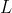be the set of all propositions generated by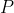and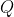(Whereandare Two Propositions) using at most one of the following logical operations :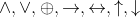, Where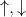are NAND and NOR operators respectively.

i.e.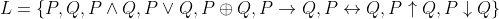A Relation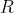is defined onsuch that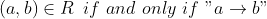is Tautology.

The relation R is

(A). Reflexive, symmetric, Transitive

(B). Reflexive, symmetric, Not transitive

(C). Reflexive, anti-symmetric, Transitive

(D). Irreflexive, asymmetric, Transitiveasked Jun 24
reshown Jun 27answered 5 days ago by (112,760 points)